# MSBSHSE Solutions For Class 9 Maths Part 2 Chapter 2 Parallel Lines

MSBSHSE Solutions For Class 9 Maths Part 2 Chapter 2 Parallel Lines gives detailed explanations to each and every solution. This chapter deals with properties of angles formed by parallel lines and its transversal. Various tests for parallelness of two lines appear in this chapter. All solutions from MSBSHSE Solutions For Class 9 Maths Part 2 Chapter 2 Parallel Lines are provided here for free. BYJU’S provides error free solutions prepared by our subject matter experts. Students can easily download these solutions in PDF format.

## Download the PDF of Maharashtra Solutions For Class 9 Maths Part 2 Chapter 2 Parallel Lines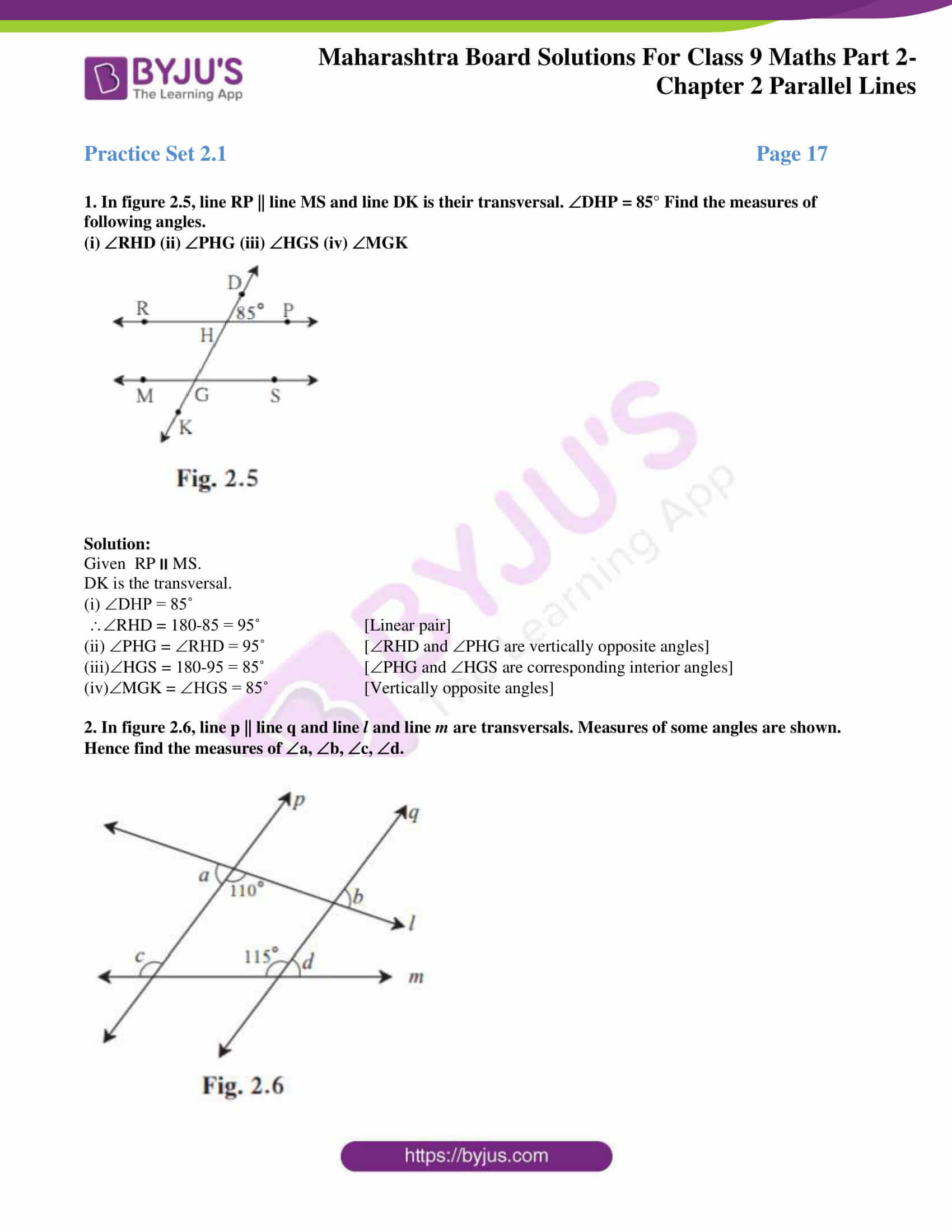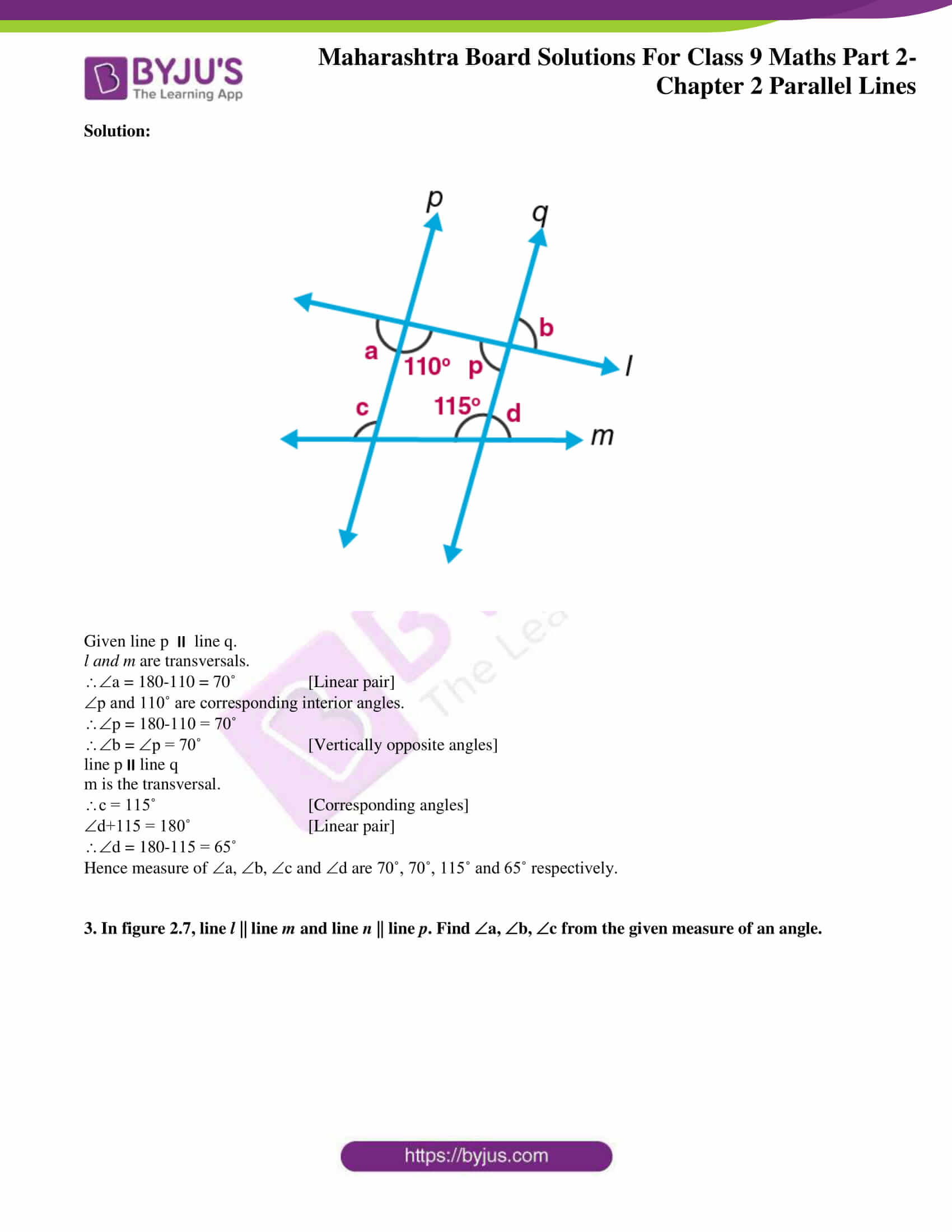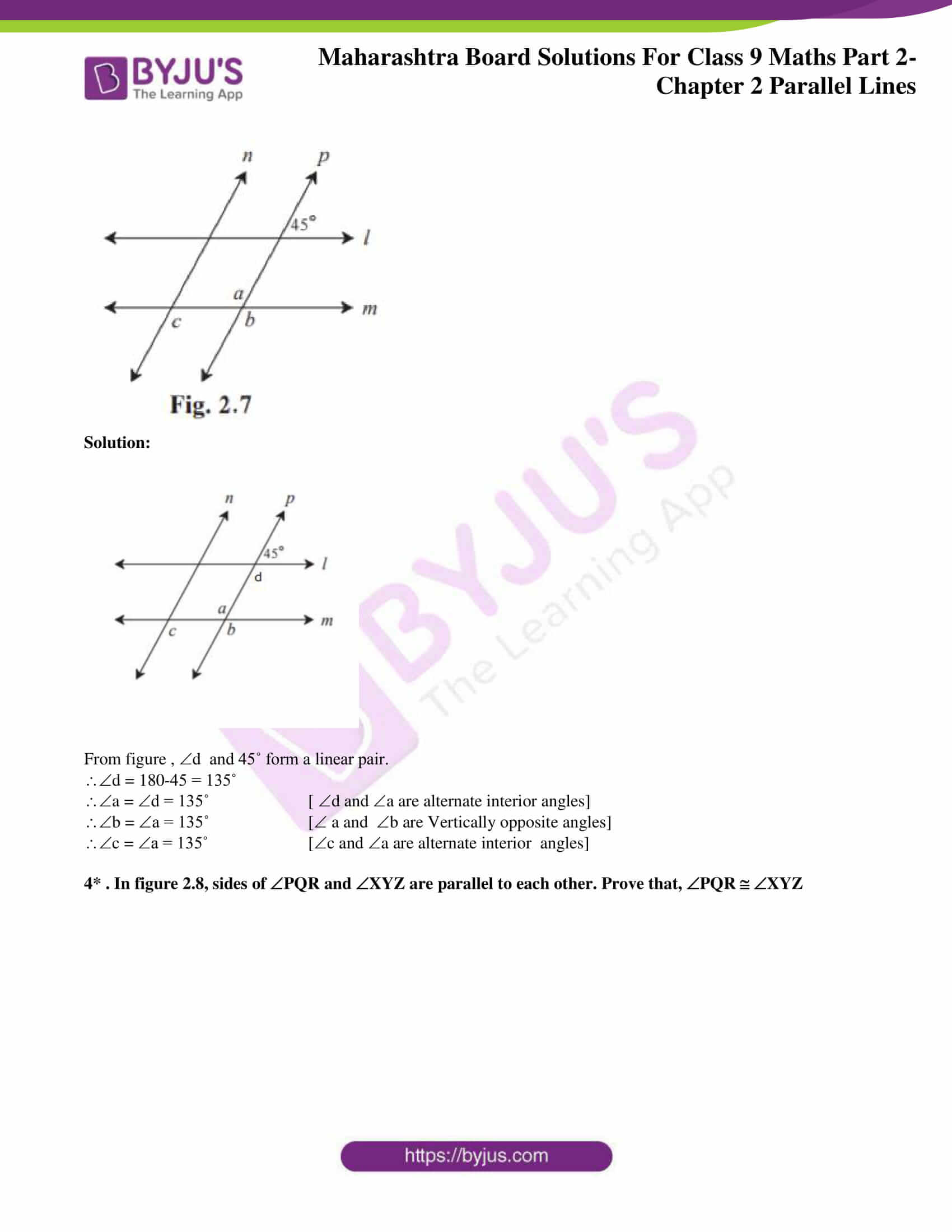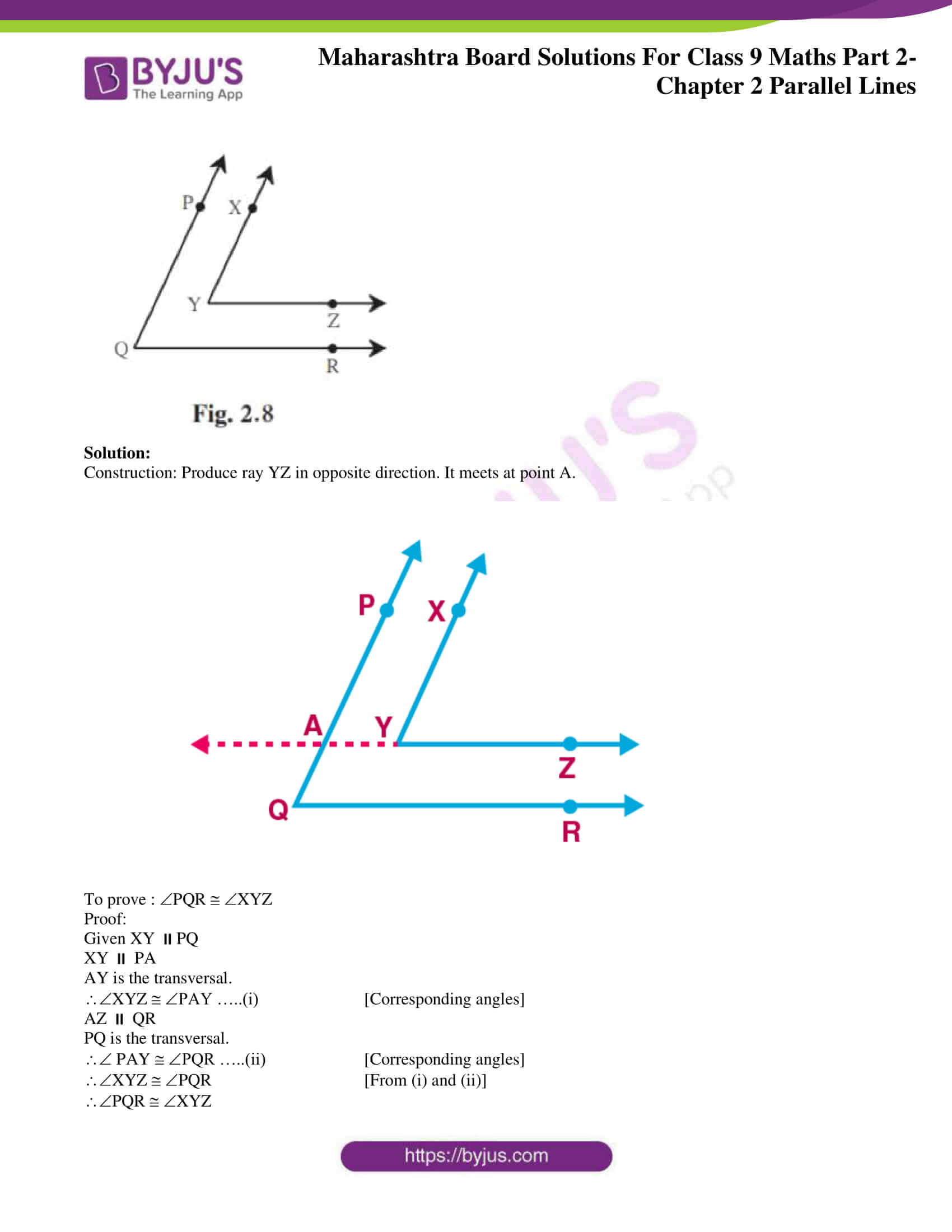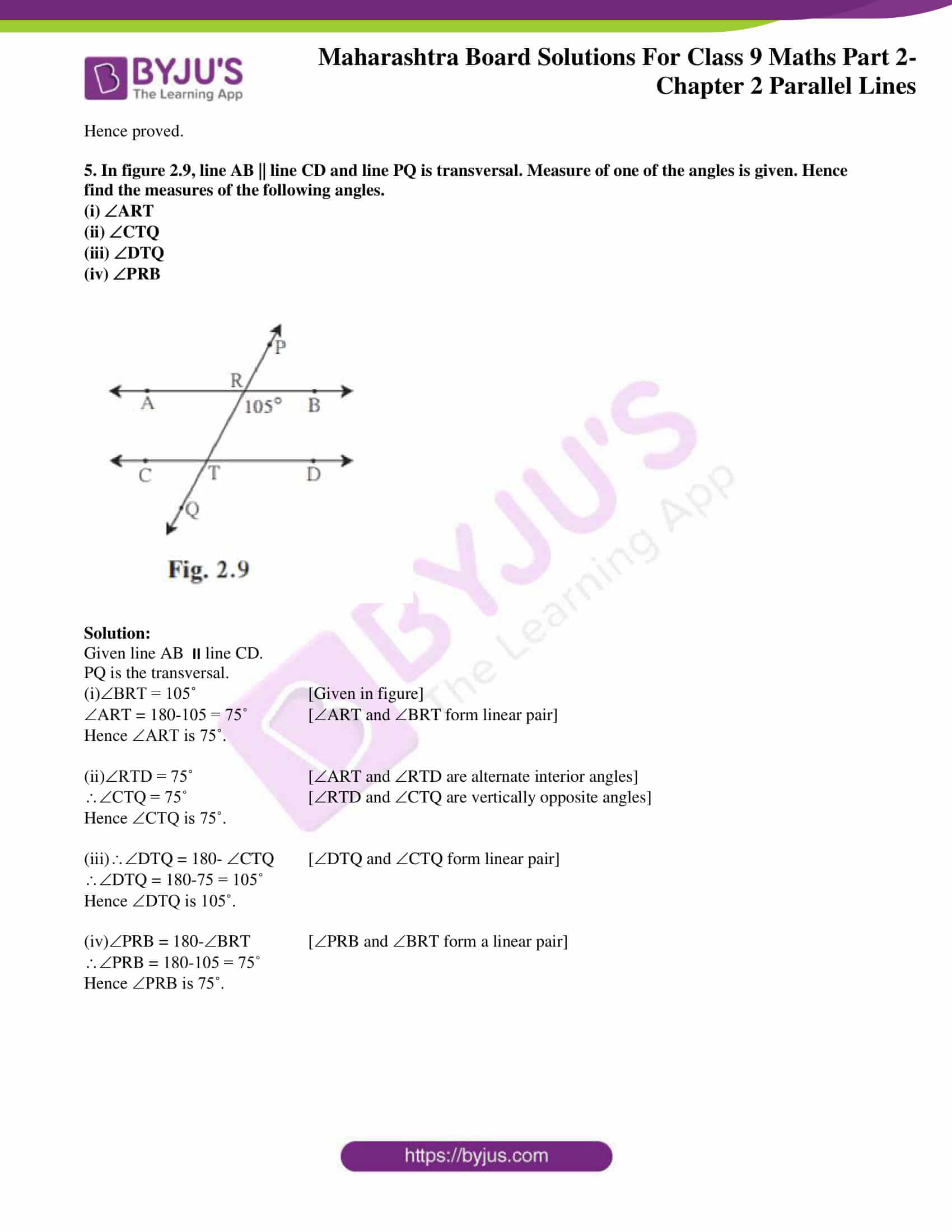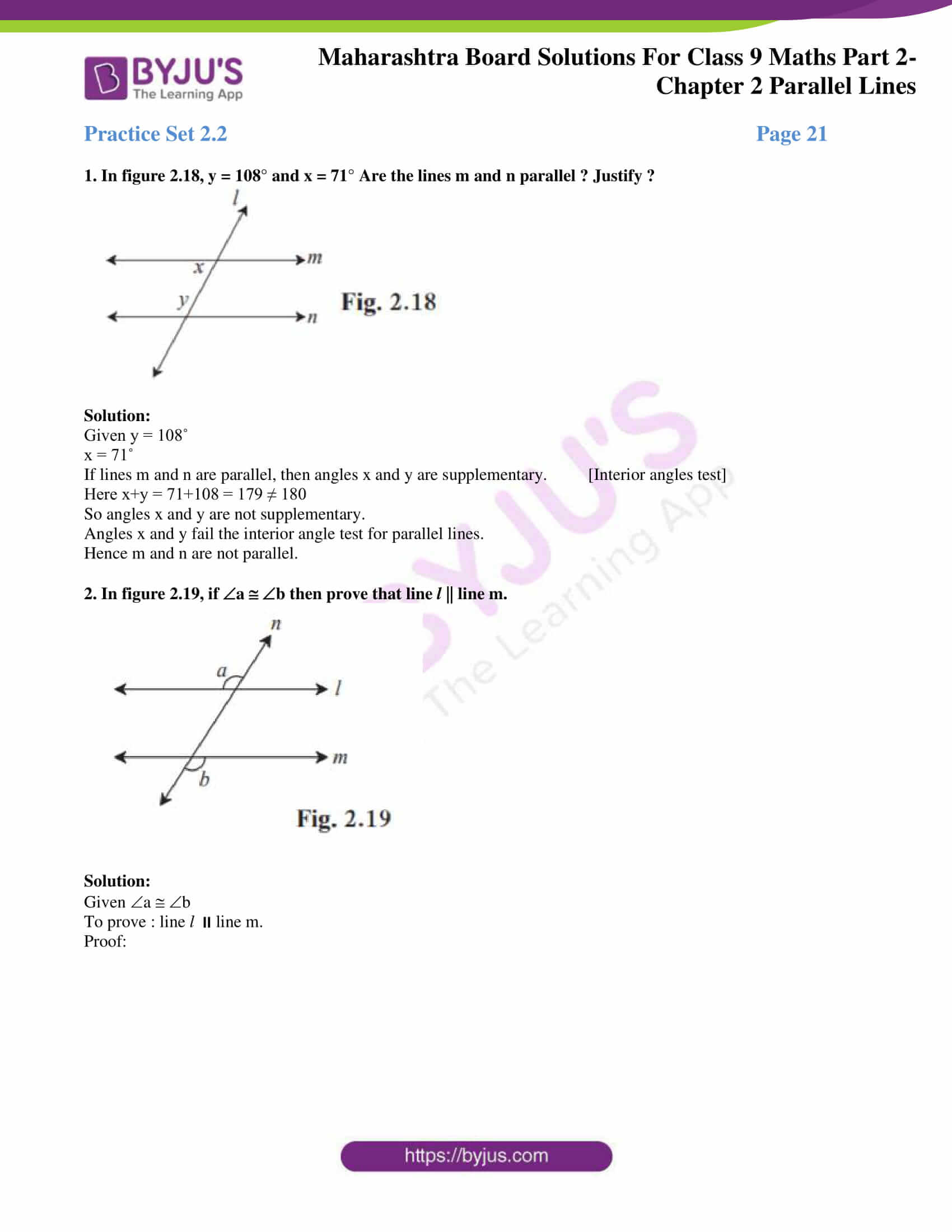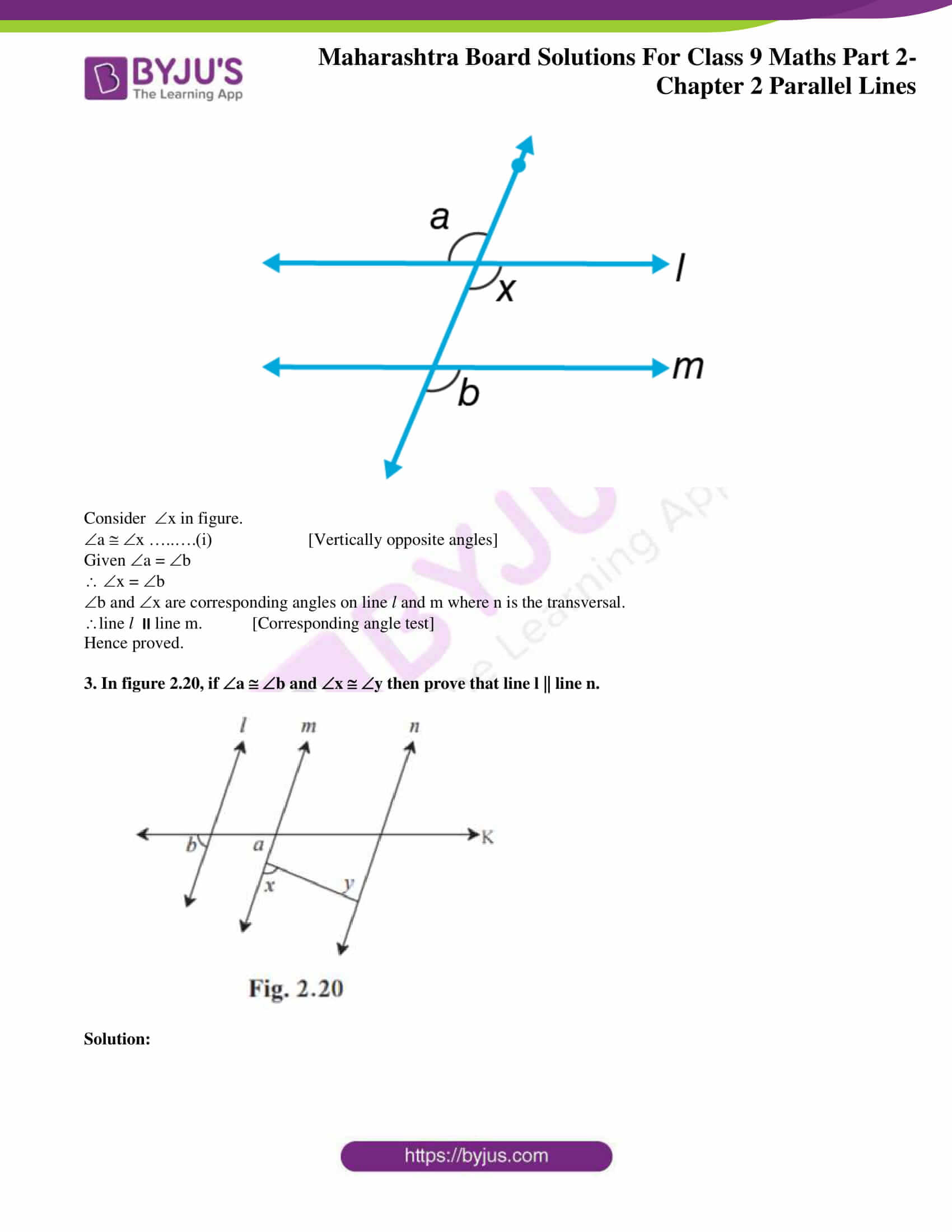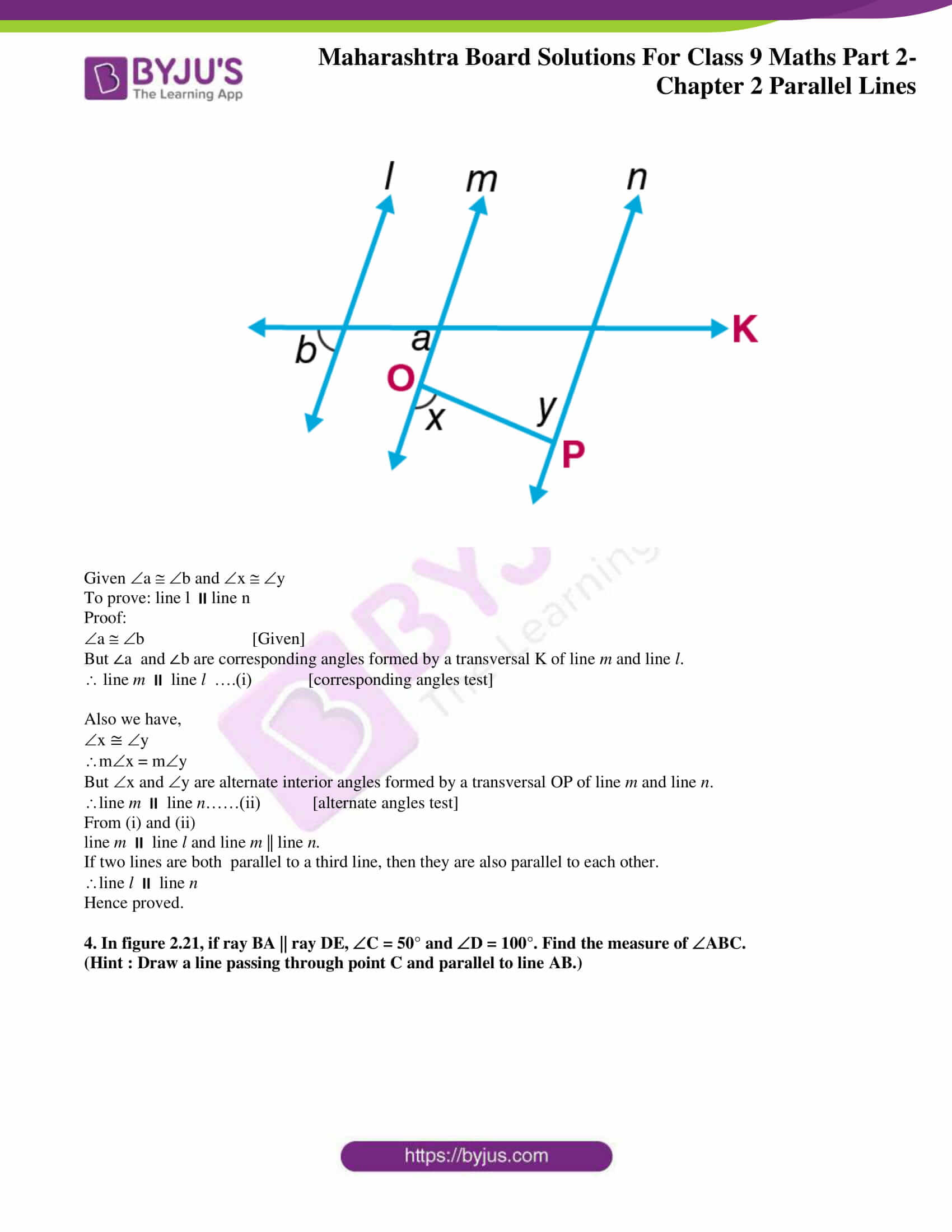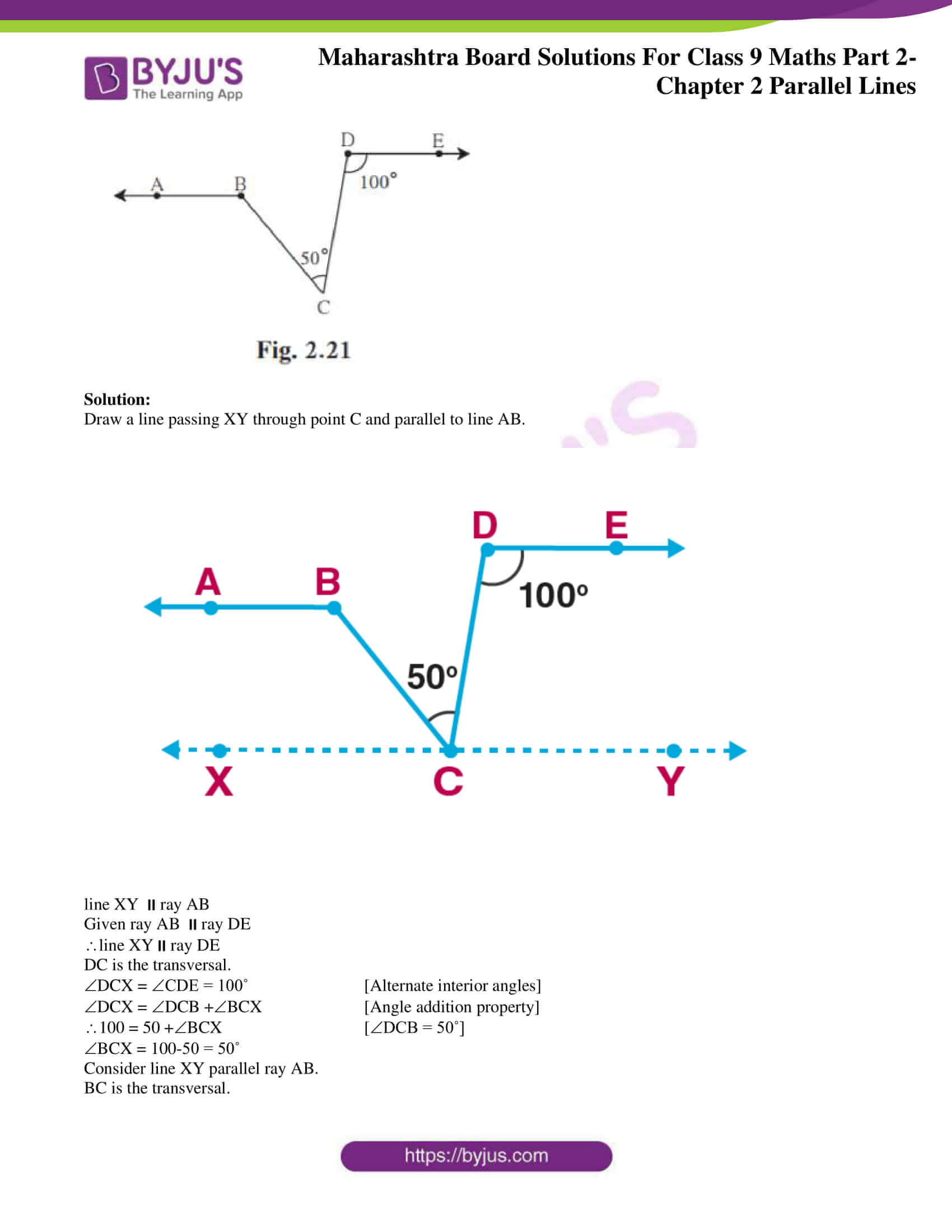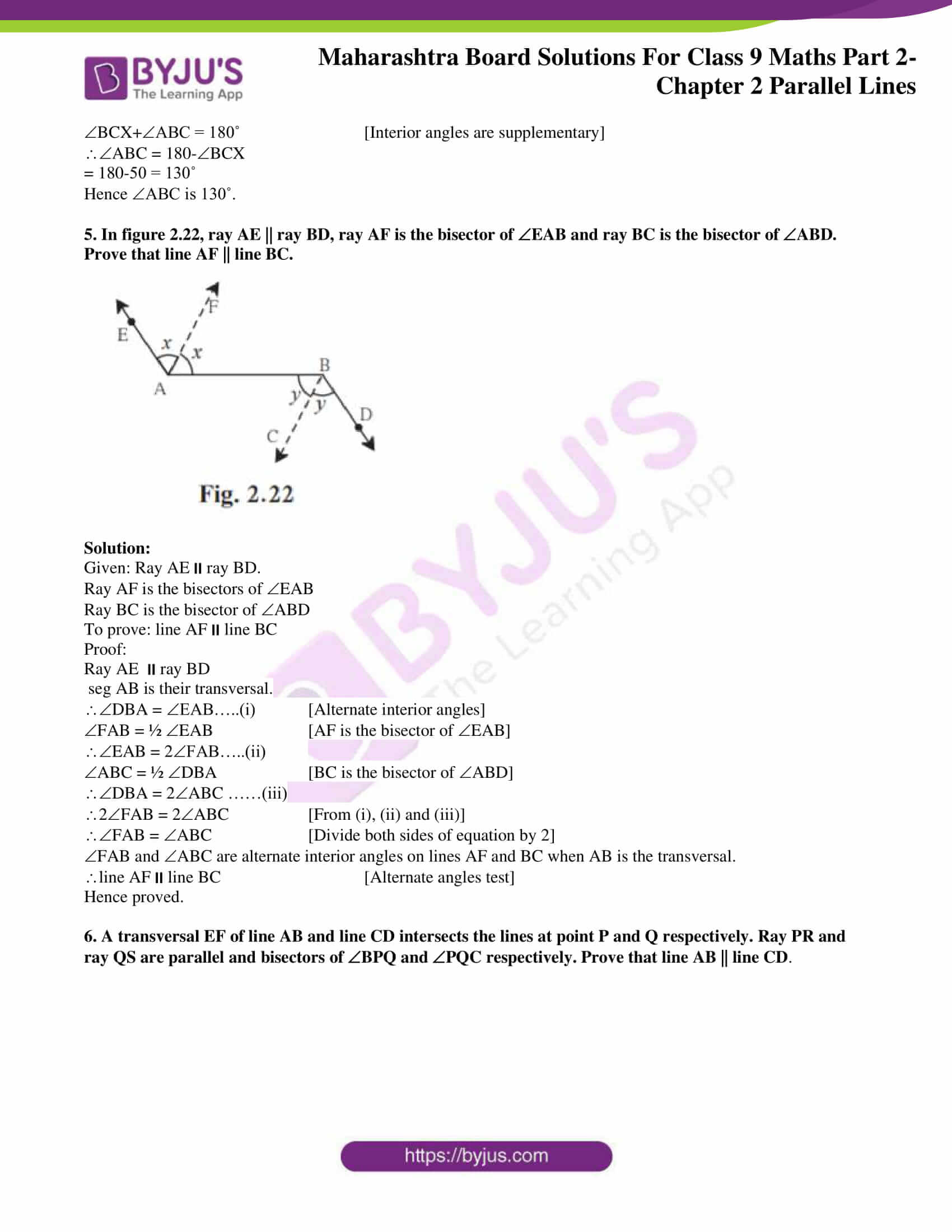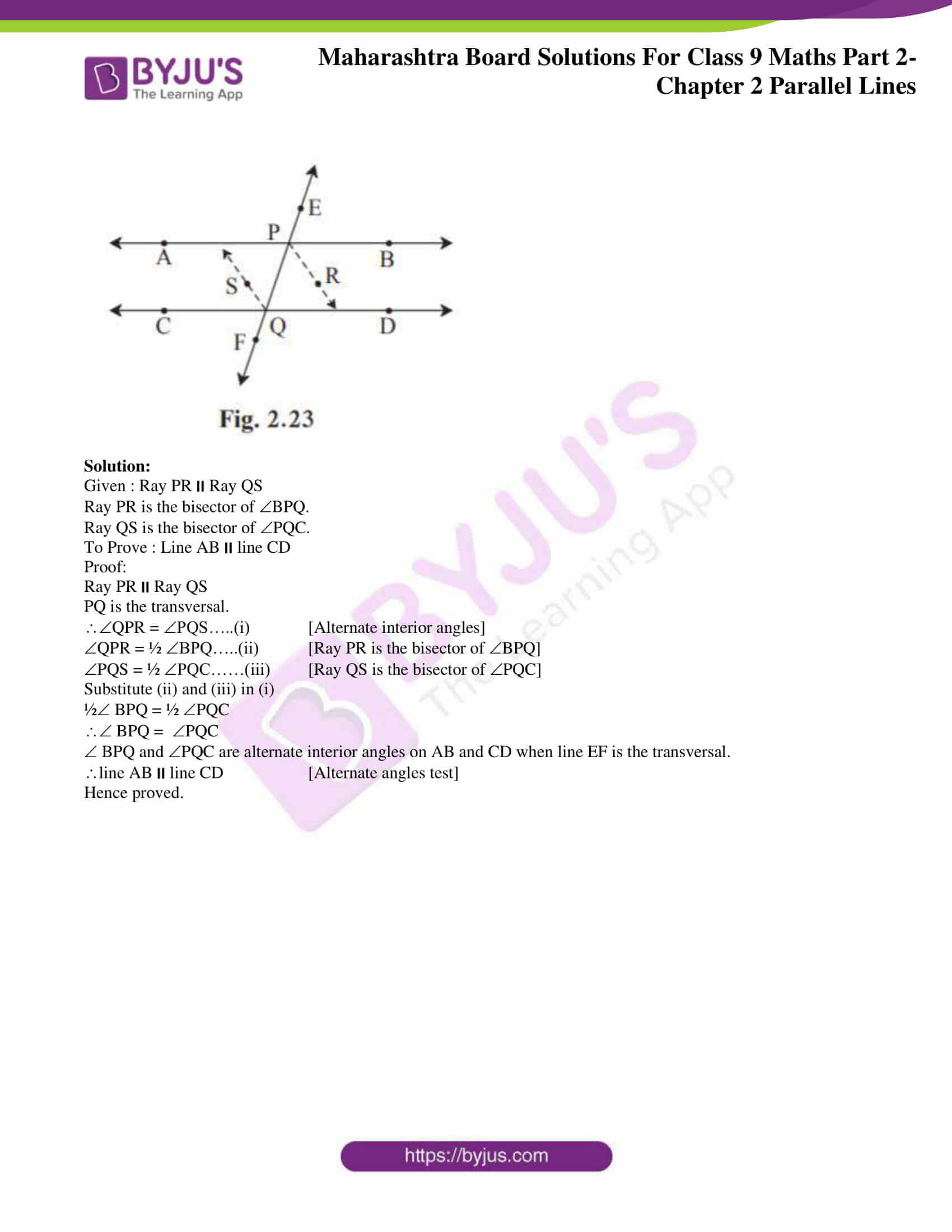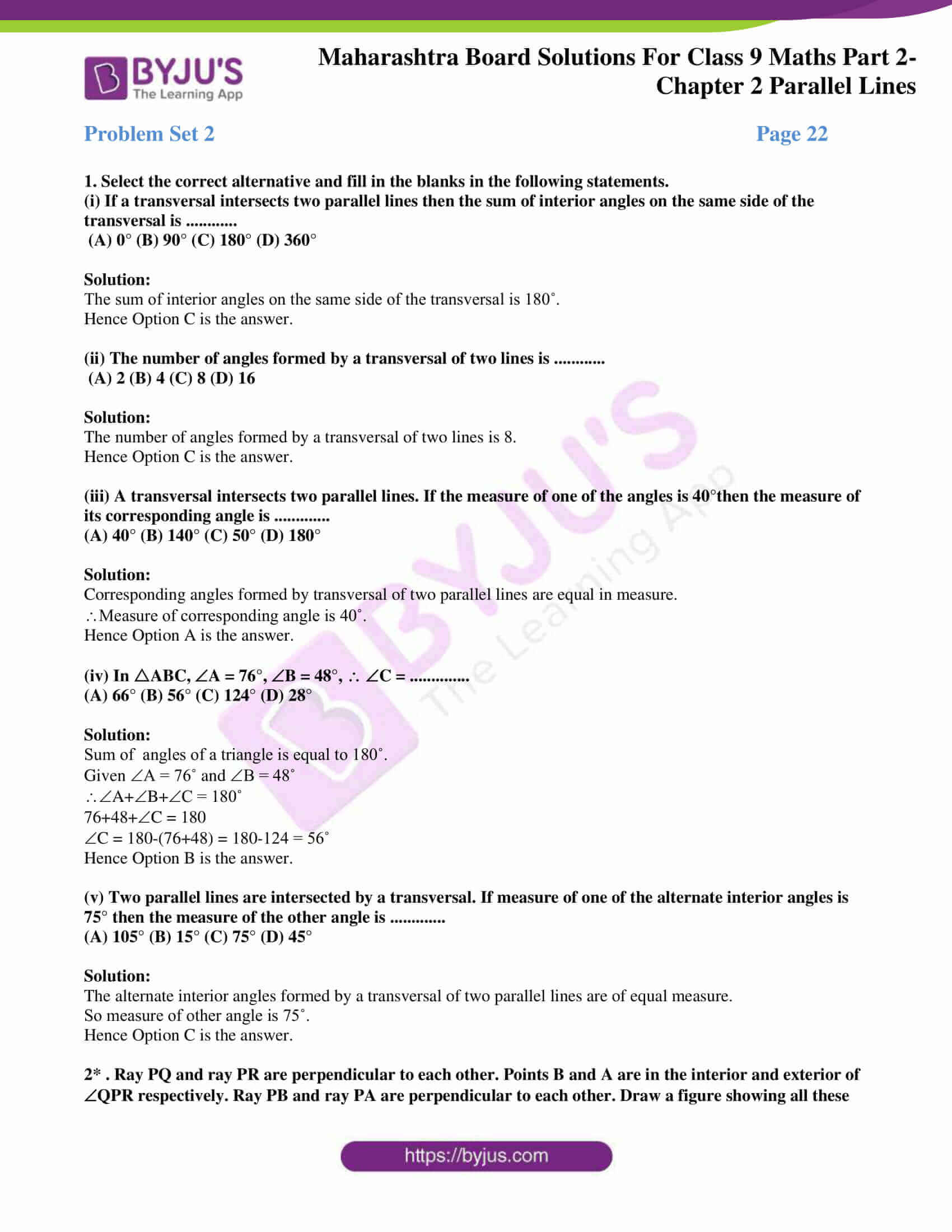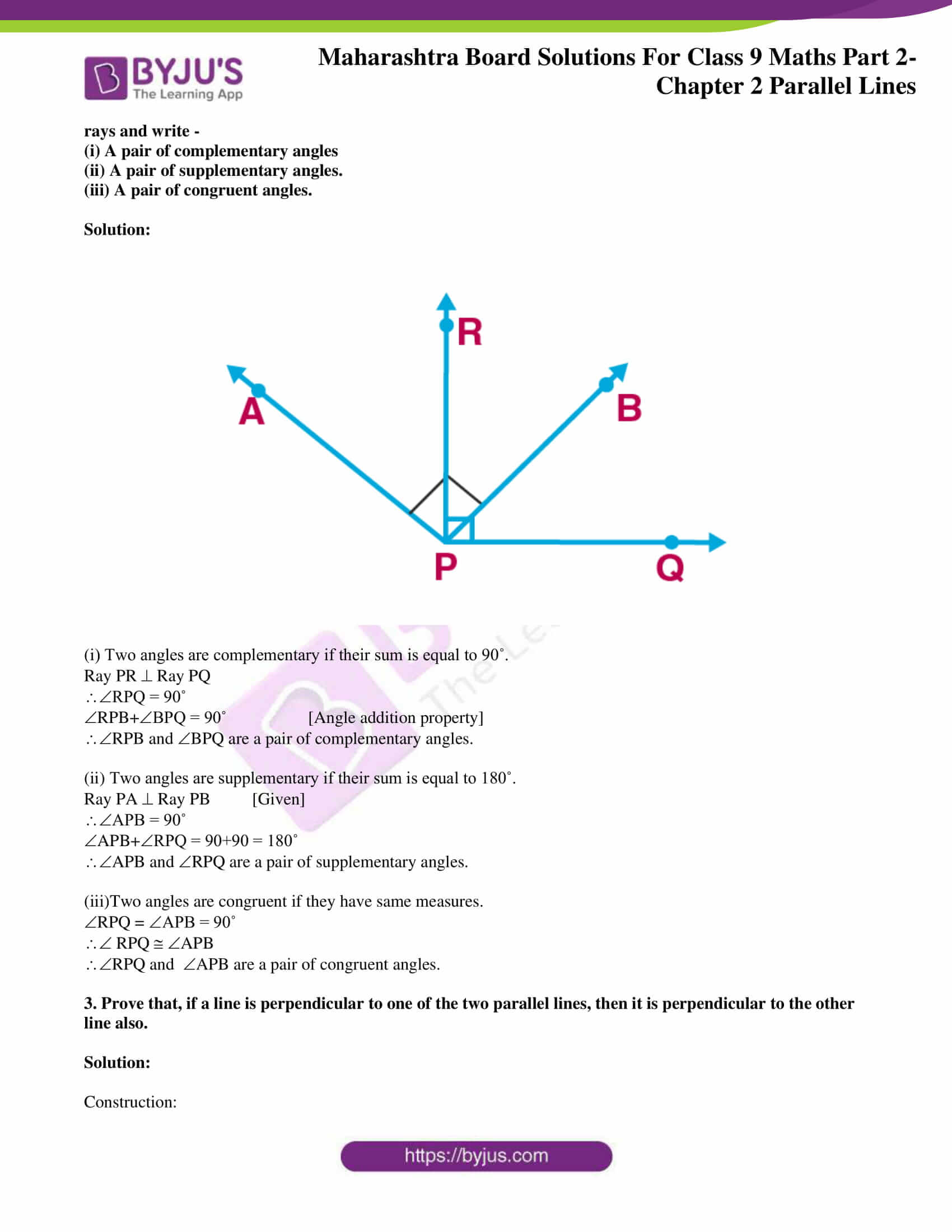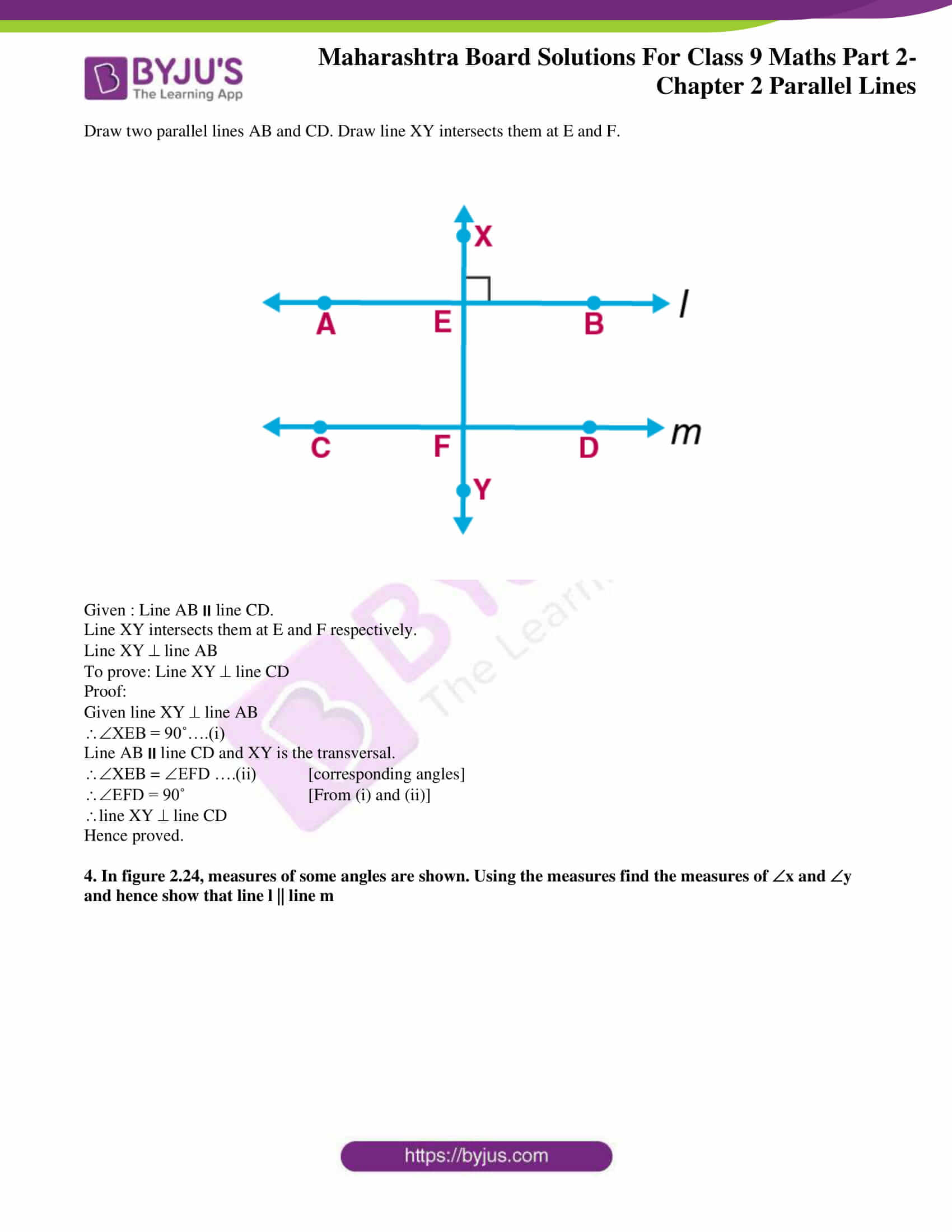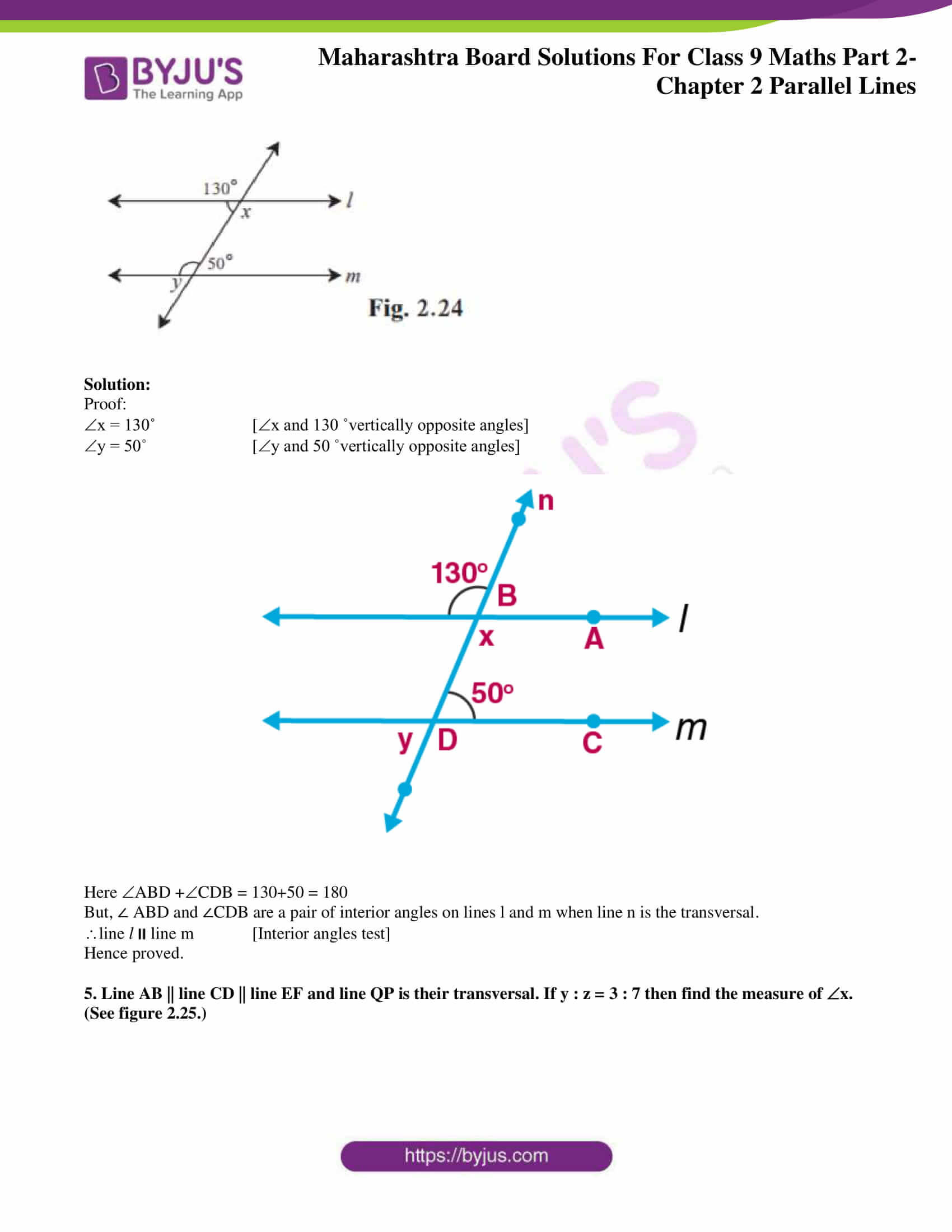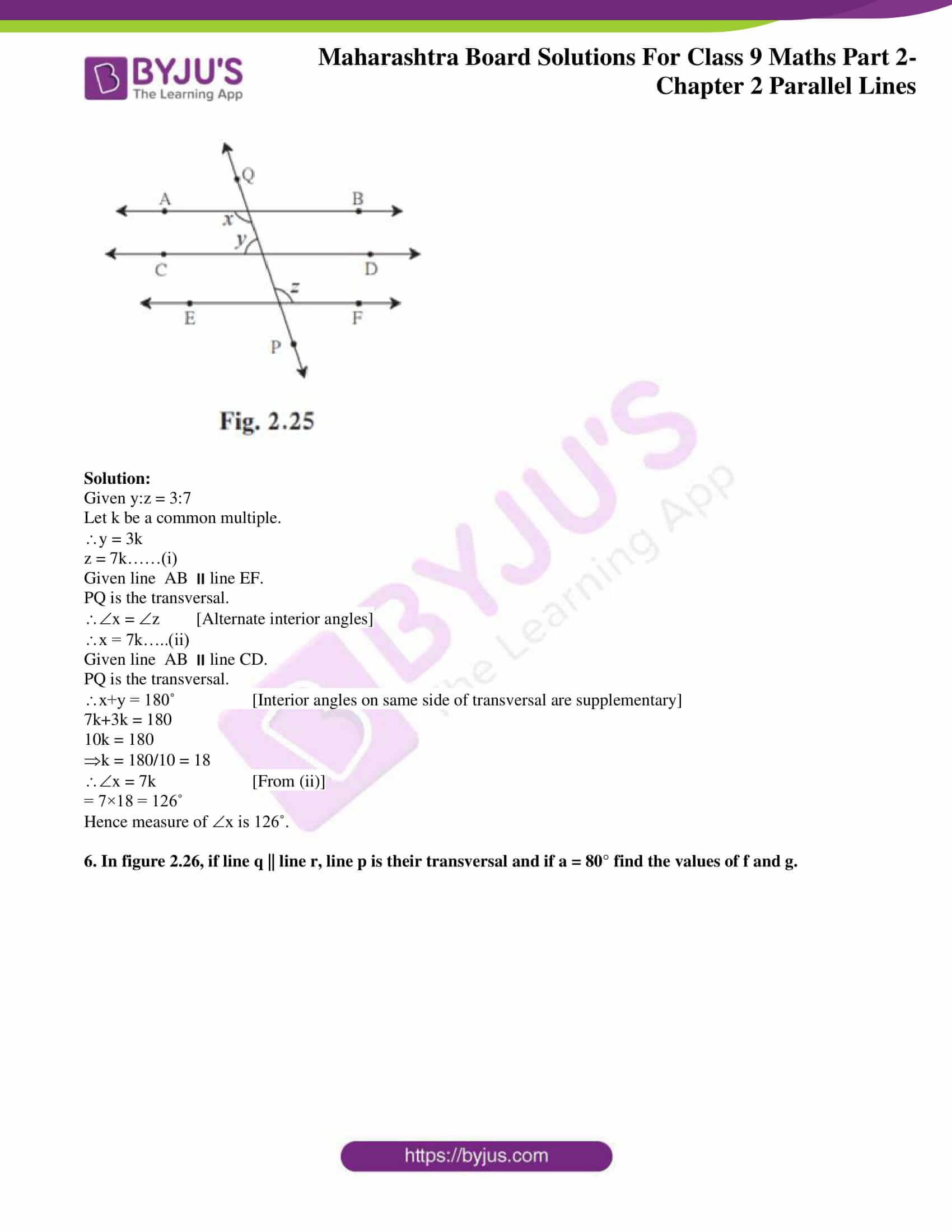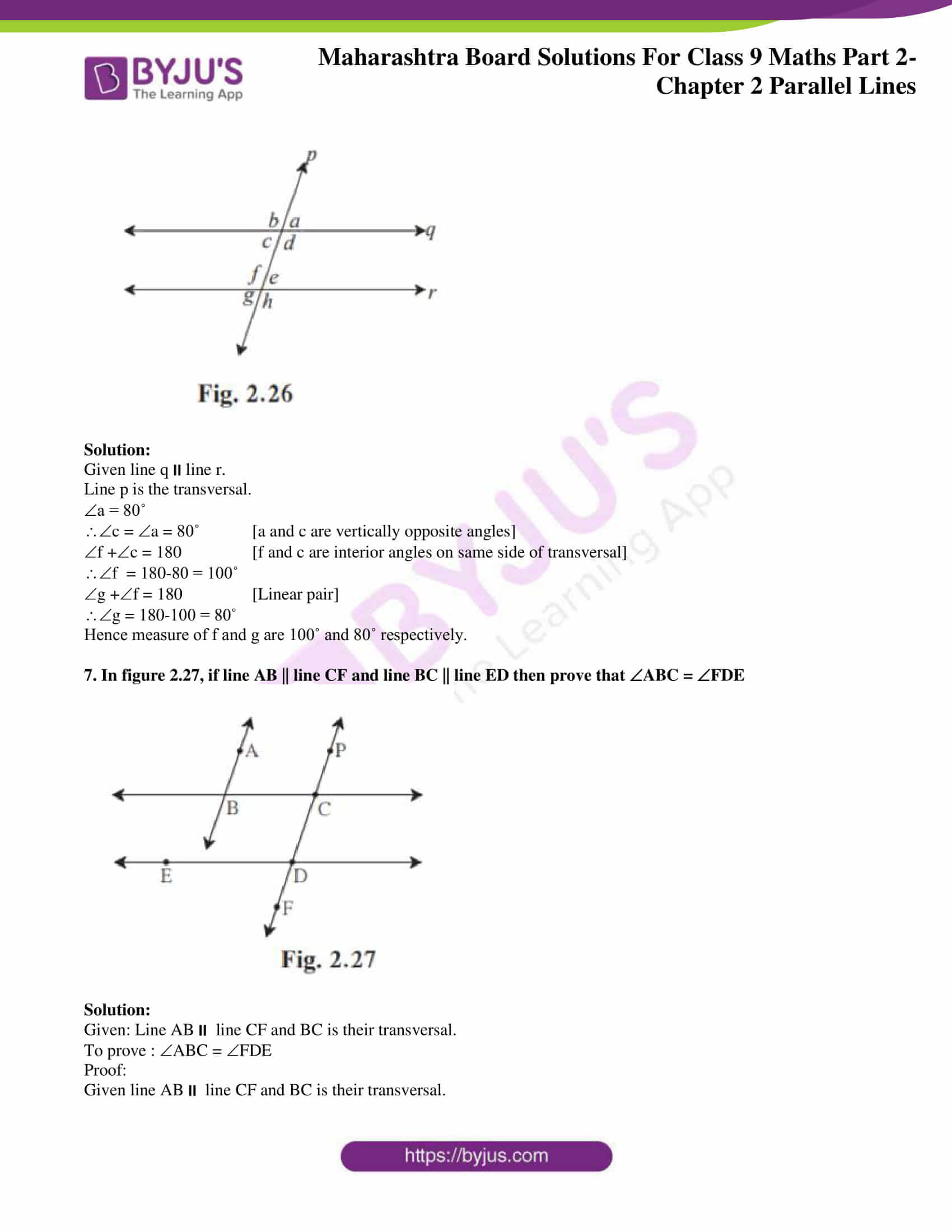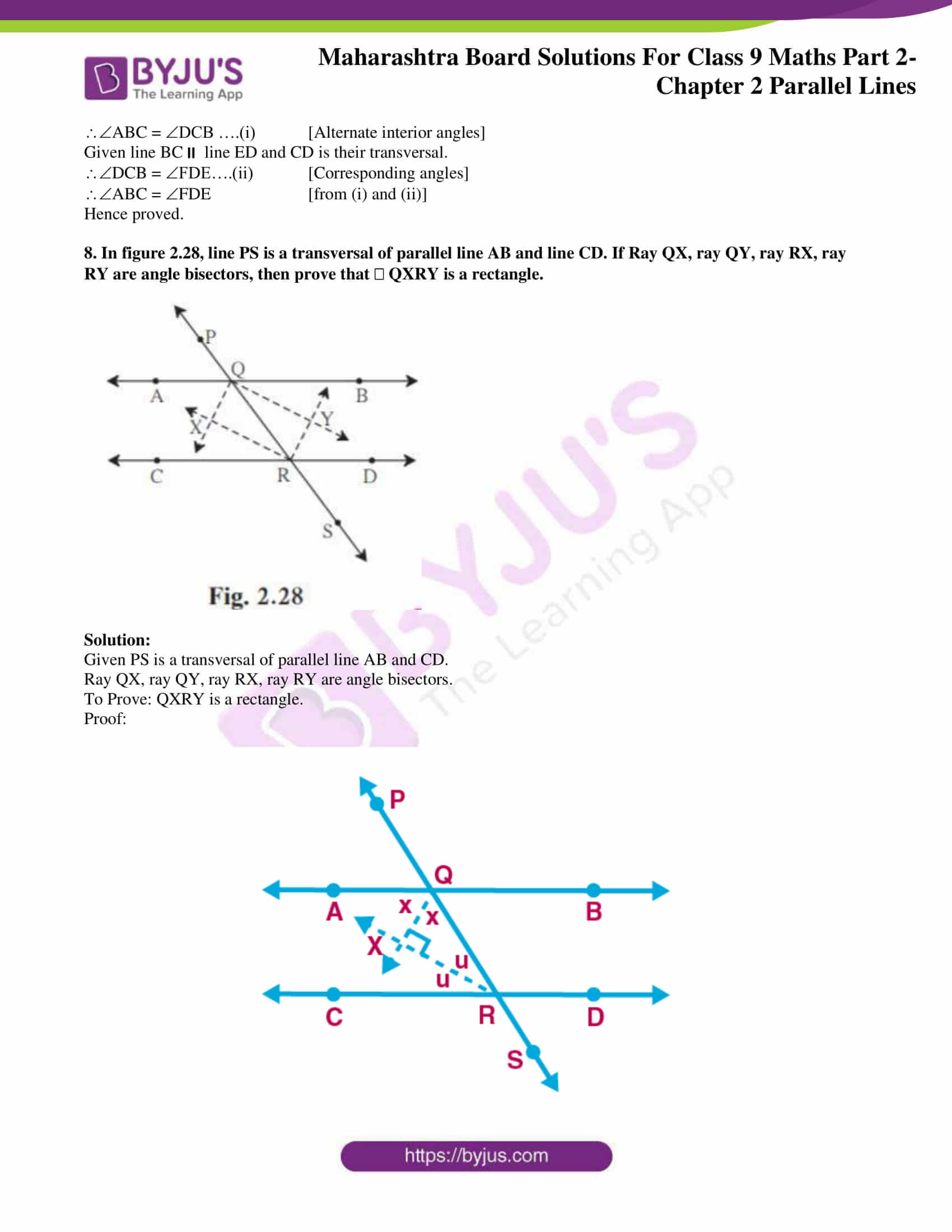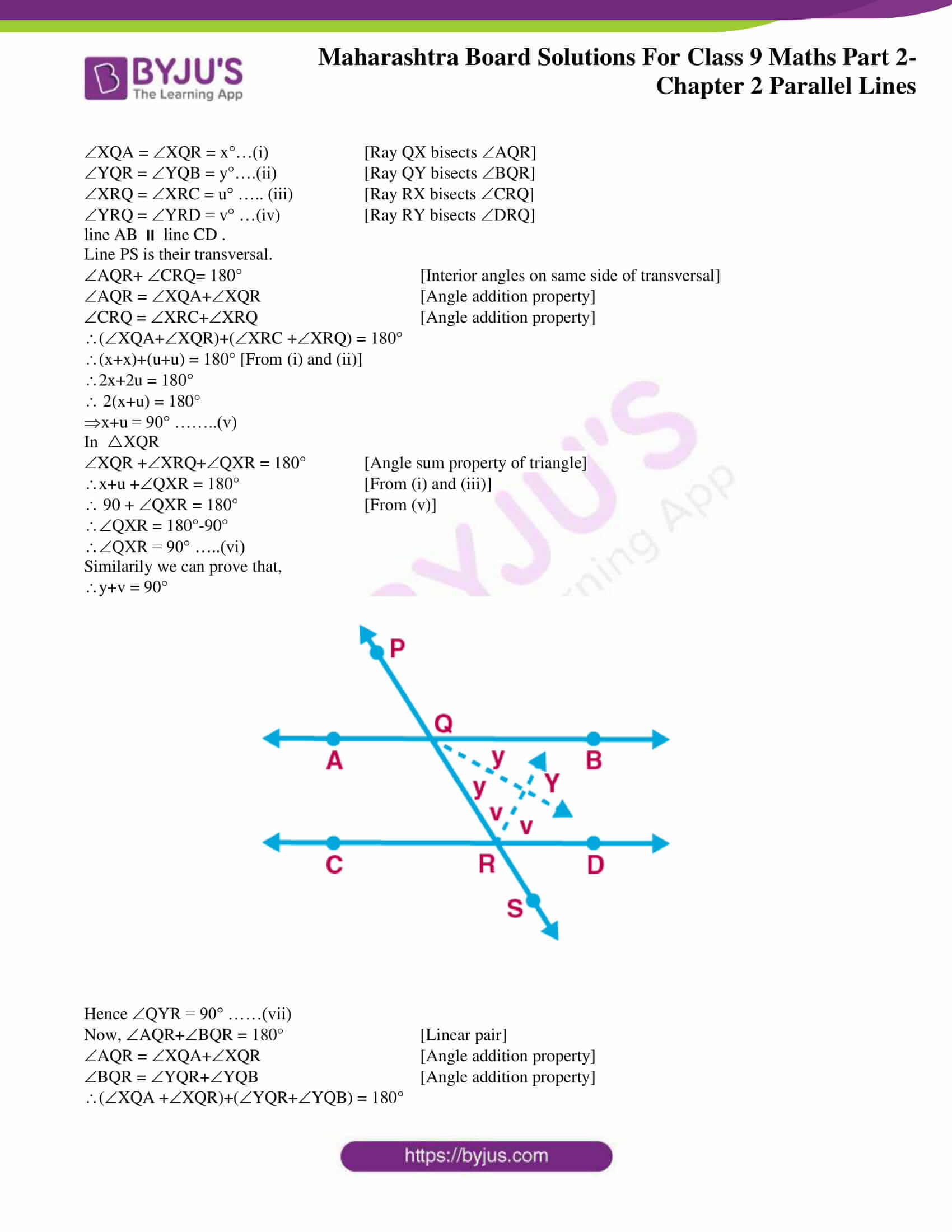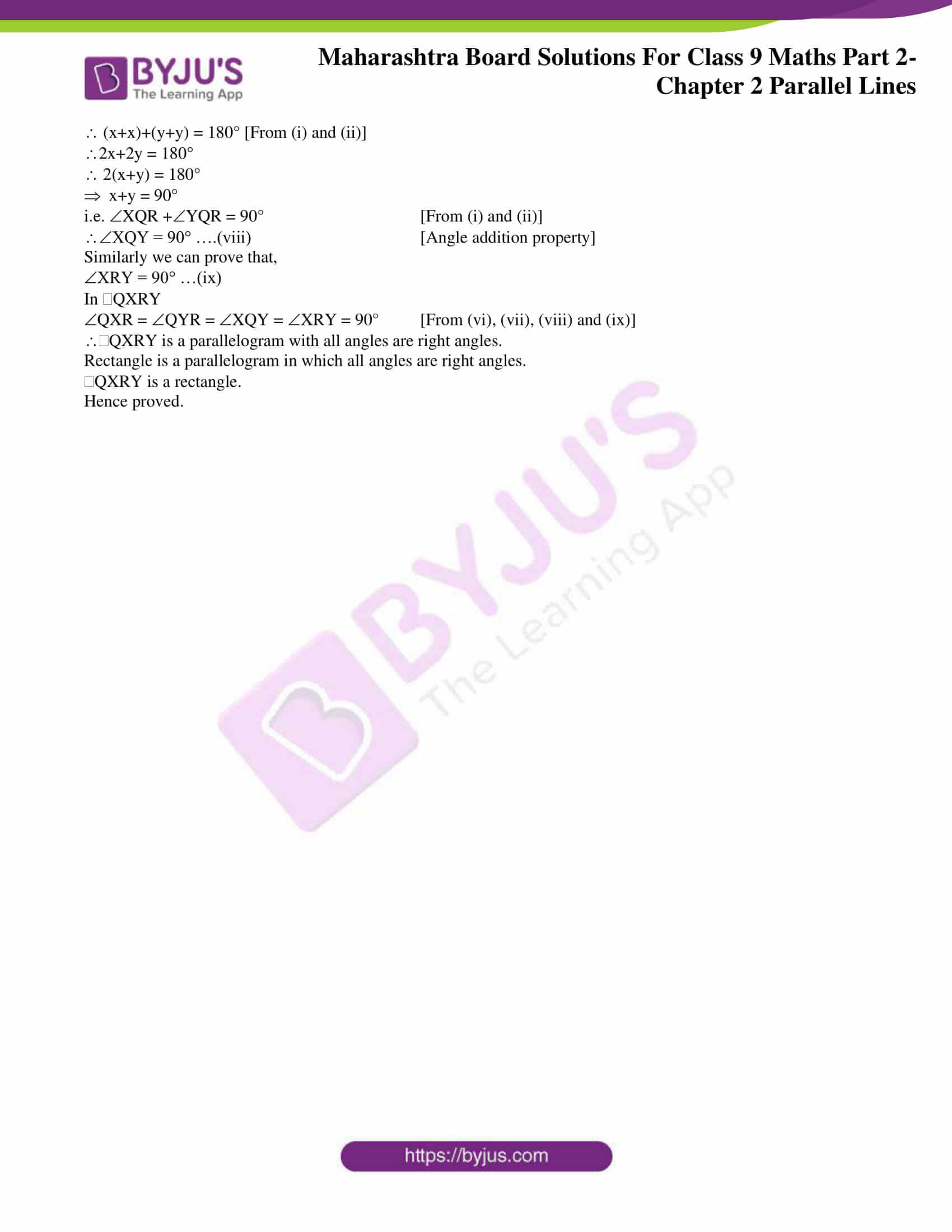### Access answers to Maths MSBSHSE Solutions For Class 9 Part 2 Chapter 2 – Parallel Lines

Practice Set 2.1 Page 17

1. In figure 2.5, line RP || line MS and line DK is their transversal. DHP = 85° Find the measures of following angles.

(i) RHD (ii) PHG (iii) HGS (iv) MGK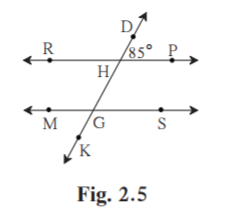Solution:

Given RPMS.

DK is the transversal.

(i) DHP = 85˚

RHD = 180-85 = 95˚ [Linear pair]

(ii) PHG = RHD = 95˚ [RHD and PHG are vertically opposite angles]

(iii)HGS = 180-95 = 85˚ [PHG and HGS are corresponding interior angles]

(iv)MGK = HGS = 85˚ [Vertically opposite angles]

2. In figure 2.6, line p || line q and line l and line m are transversals. Measures of some angles are shown. Hence find the measures of a, b, c, d.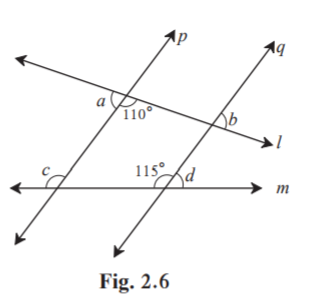Solution: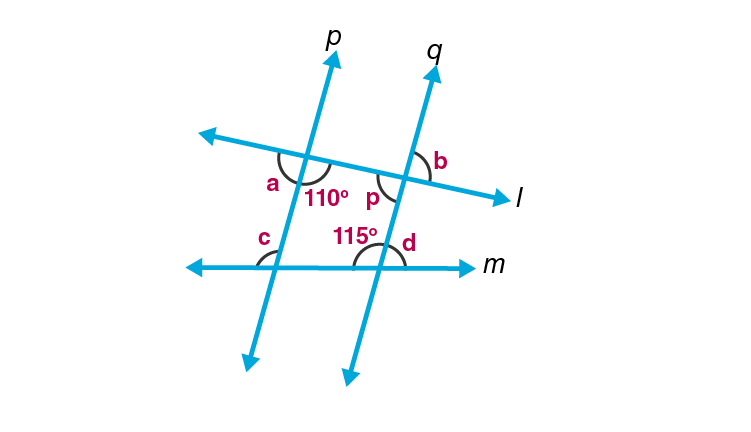Given line p line q.

l and m are transversals.

a = 180-110 = 70˚ [Linear pair]

p and 110˚ are corresponding interior angles.

p = 180-110 = 70˚

b = p = 70˚ [Vertically opposite angles]

line pline q

m is the transversal.

c = 115˚ [Corresponding angles]

d+115 = 180˚ [Linear pair]

d = 180-115 = 65˚

Hence measure of a, b, c and d are 70˚, 70˚, 115˚ and 65˚ respectively.

3. In figure 2.7, line l || line m and line n || line p. Find a, b, c from the given measure of an angle.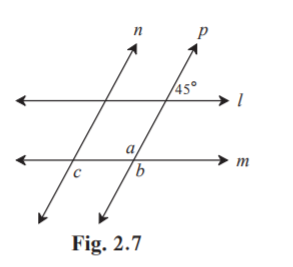Solution: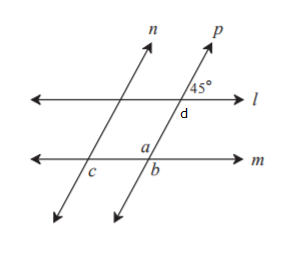From figure , d and 45˚ form a linear pair.

d = 180-45 = 135˚

a = d = 135˚ [ d and a are alternate interior angles]

b = a = 135˚ [ a and b are Vertically opposite angles]

c = a = 135˚ [c and a are alternate interior angles]

4* . In figure 2.8, sides of PQR and XYZ are parallel to each other. Prove that, PQR XYZ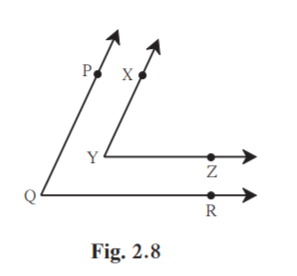Solution:

Construction: Produce ray YZ in opposite direction. It meets at point A.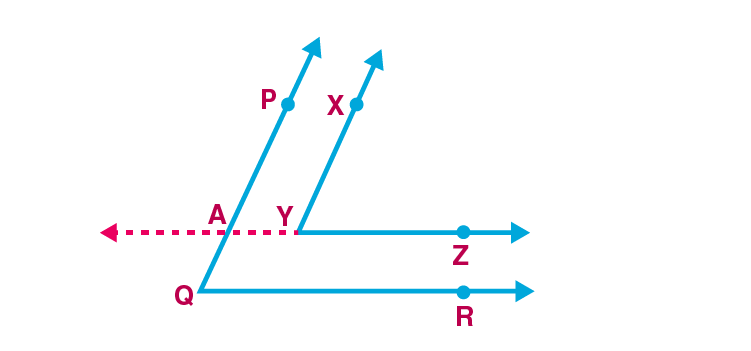To prove : PQR XYZ

Proof:

Given XY PQ

XY PA

AY is the transversal.

XYZ PAY …..(i) [Corresponding angles]

AZ QR

PQ is the transversal.

PAY PQR …..(ii) [Corresponding angles]

XYZ PQR [From (i) and (ii)]

PQR XYZ

Hence proved.

5. In figure 2.9, line AB || line CD and line PQ is transversal. Measure of one of the angles is given. Hence find the measures of the following angles.

(i) ART

(ii) CTQ

(iii) DTQ

(iv) PRB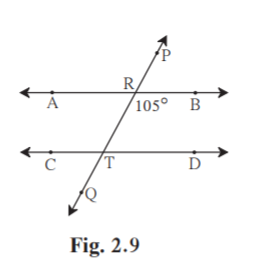Solution:

Given line AB line CD.

PQ is the transversal.

(i)BRT = 105˚ [Given in figure]

ART = 180-105 = 75˚ [ART and BRT form linear pair]

Hence ART is 75˚.

(ii)RTD = 75˚ [ART and RTD are alternate interior angles]

CTQ = 75˚ [RTD and CTQ are vertically opposite angles]

Hence CTQ is 75˚.

(iii)DTQ = 180- CTQ [DTQ and CTQ form linear pair]

DTQ = 180-75 = 105˚

Hence DTQ is 105˚.

(iv)PRB = 180-BRT [PRB and BRT form a linear pair]

PRB = 180-105 = 75˚

Hence PRB is 75˚.

Practice Set 2.2 Page 21

1. In figure 2.18, y = 108° and x = 71° Are the lines m and n parallel ? Justify ?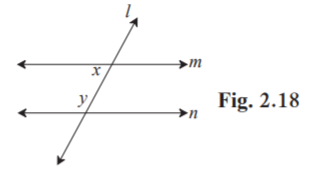Solution:

Given y = 108˚

x = 71˚

If lines m and n are parallel, then angles x and y are supplementary. [Interior angles test]

Here x+y = 71+108 = 179 ≠ 180

So angles x and y are not supplementary.

Angles x and y fail the interior angle test for parallel lines.

Hence m and n are not parallel.

2. In figure 2.19, if a b then prove that line l || line m.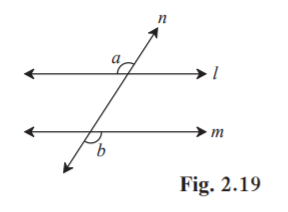Solution:

Given a b

To prove : line l line m.

Proof: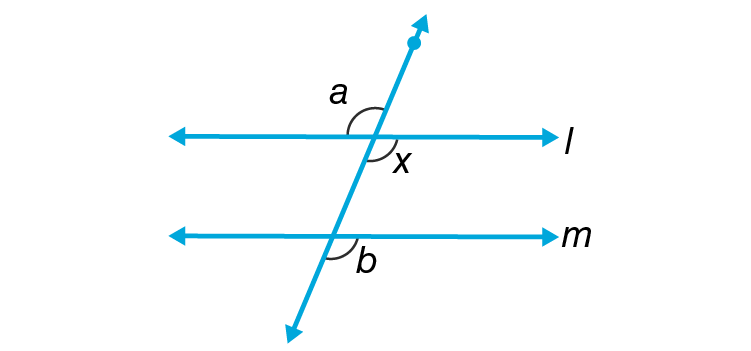Consider x in figure.

a x …..….(i) [Vertically opposite angles]

Given a = b

x = b

b and x are corresponding angles on line l and m where n is the transversal.

line l line m. [Corresponding angle test]

Hence proved.

3. In figure 2.20, if a b and x y then prove that line l || line n.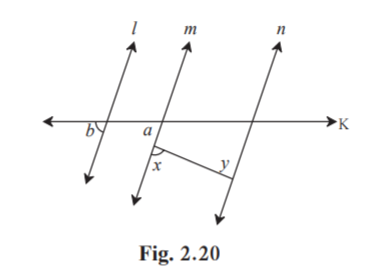Solution: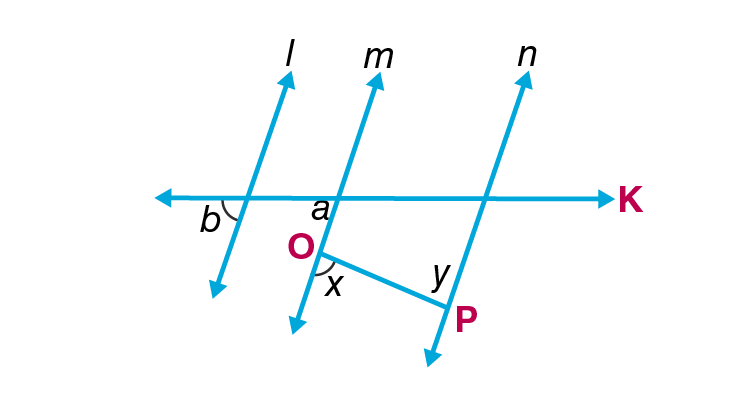Given a b and x y

To prove: line l line n

Proof:

a b [Given]

But ∠a  and ∠b are corresponding angles formed by a transversal K of line m and line l.

line m  line l  ….(i)  [corresponding angles test]

Also we have,

x ≅ y

mx = my

But x and y are alternate interior angles formed by a transversal OP of line m and line n.

line m  line n……(ii)   [alternate angles test]

From (i) and (ii)

line m  line l and line m || line n.

If two lines are both parallel to a third line, then they are also parallel to each other.

line l  line n

Hence proved.

4. In figure 2.21, if ray BA || ray DE, C = 50° and D = 100°. Find the measure of ABC.

(Hint : Draw a line passing through point C and parallel to line AB.)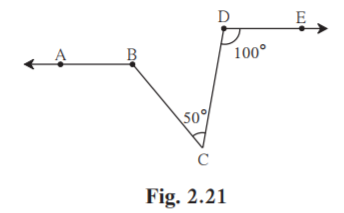Solution:

Draw a line passing XY through point C and parallel to line AB.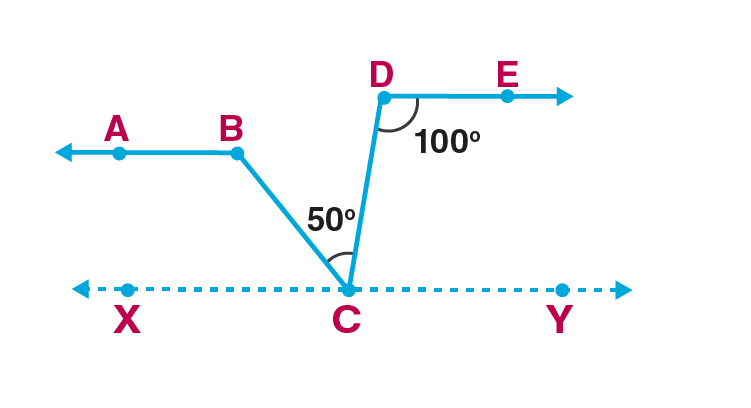line XY ray AB

Given ray AB ray DE

line XYray DE

DC is the transversal.

DCX = CDE = 100˚ [Alternate interior angles]

DCX = DCB +BCX [Angle addition property]

100 = 50 +BCX [DCB = 50˚]

BCX = 100-50 = 50˚

Consider line XY parallel ray AB.

BC is the transversal.

BCX+ABC = 180˚ [Interior angles are supplementary]

ABC = 180-BCX

= 180-50 = 130˚

Hence ABC is 130˚.

5. In figure 2.22, ray AE || ray BD, ray AF is the bisector of EAB and ray BC is the bisector of ABD. Prove that line AF || line BC.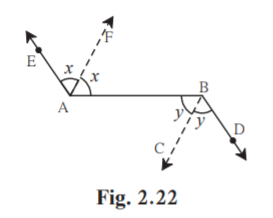Solution:

Given: Ray AEray BD.

Ray AF is the bisectors of EAB

Ray BC is the bisector of ABD

To prove: line AFline BC

Proof:

Ray AE ray BD

seg AB is their transversal.

DBA = EAB…..(i) [Alternate interior angles]

FAB = ½ EAB [AF is the bisector of EAB]

EAB = 2FAB…..(ii)

ABC = ½ DBA [BC is the bisector of ABD]

DBA = 2ABC ……(iii)

2FAB = 2ABC [From (i), (ii) and (iii)]

FAB = ABC [Divide both sides of equation by 2]

FAB and ABC are alternate interior angles on lines AF and BC when AB is the transversal.

line AFline BC [Alternate angles test]

Hence proved.

6. A transversal EF of line AB and line CD intersects the lines at point P and Q respectively. Ray PR and ray QS are parallel and bisectors of BPQ and PQC respectively. Prove that line AB || line CD.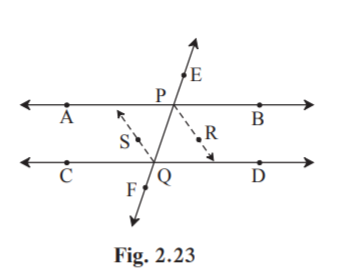Solution:

Given : Ray PRRay QS

Ray PR is the bisector of BPQ.

Ray QS is the bisector of PQC.

To Prove : Line ABline CD

Proof:

Ray PRRay QS

PQ is the transversal.

QPR = PQS…..(i) [Alternate interior angles]

QPR = ½ BPQ…..(ii) [Ray PR is the bisector of BPQ]

PQS = ½ PQC……(iii) [Ray QS is the bisector of PQC]

Substitute (ii) and (iii) in (i)

½ BPQ = ½ PQC

BPQ = PQC

BPQ and PQC are alternate interior angles on AB and CD when line EF is the transversal.

line ABline CD [Alternate angles test]

Hence proved.

Problem Set 2 Page 22

1. Select the correct alternative and fill in the blanks in the following statements.

(i) If a transversal intersects two parallel lines then the sum of interior angles on the same side of the

transversal is …………

(A) 0° (B) 90° (C) 180° (D) 360°

Solution:

The sum of interior angles on the same side of the transversal is 180˚.

Hence Option C is the answer.

(ii) The number of angles formed by a transversal of two lines is …………

(A) 2 (B) 4 (C) 8 (D) 16

Solution:

The number of angles formed by a transversal of two lines is 8.

Hence Option C is the answer.

(iii) A transversal intersects two parallel lines. If the measure of one of the angles is 40°then the measure of its corresponding angle is ………….

(A) 40° (B) 140° (C) 50° (D) 180°

Solution:

Corresponding angles formed by transversal of two parallel lines are equal in measure.

Measure of corresponding angle is 40˚.

Hence Option A is the answer.

(iv) In ABC, A = 76°, B = 48°, C = …………..

(A) 66° (B) 56° (C) 124° (D) 28°

Solution:

Sum of angles of a triangle is equal to 180˚.

Given A = 76˚ and B = 48˚

A+B+C = 180˚

76+48+C = 180

C = 180-(76+48) = 180-124 = 56˚

Hence Option B is the answer.

(v) Two parallel lines are intersected by a transversal. If measure of one of the alternate interior angles is 75° then the measure of the other angle is ………….

(A) 105° (B) 15° (C) 75° (D) 45°

Solution:

The alternate interior angles formed by a transversal of two parallel lines are of equal measure.

So measure of other angle is 75˚.

Hence Option C is the answer.

2* . Ray PQ and ray PR are perpendicular to each other. Points B and A are in the interior and exterior of QPR respectively. Ray PB and ray PA are perpendicular to each other. Draw a figure showing all these rays and write –

(i) A pair of complementary angles

(ii) A pair of supplementary angles.

(iii) A pair of congruent angles.

Solution: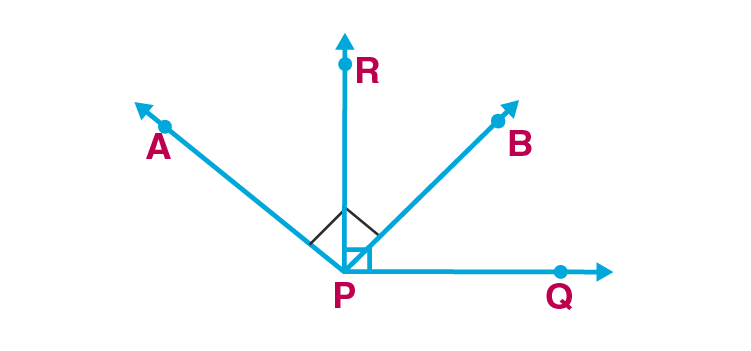(i) Two angles are complementary if their sum is equal to 90˚.

Ray PR Ray PQ

RPQ = 90˚

RPB+BPQ = 90˚ [Angle addition property]

RPB and BPQ are a pair of complementary angles.

(ii) Two angles are supplementary if their sum is equal to 180˚.

Ray PA Ray PB [Given]

APB = 90˚

APB+RPQ = 90+90 = 180˚

APB and RPQ are a pair of supplementary angles.

(iii)Two angles are congruent if they have same measures.

RPQ = APB = 90˚

RPQ APB

RPQ and APB are a pair of congruent angles.

3. Prove that, if a line is perpendicular to one of the two parallel lines, then it is perpendicular to the other line also.

Solution:

Construction:

Draw two parallel lines AB and CD. Draw line XY intersects them at E and F.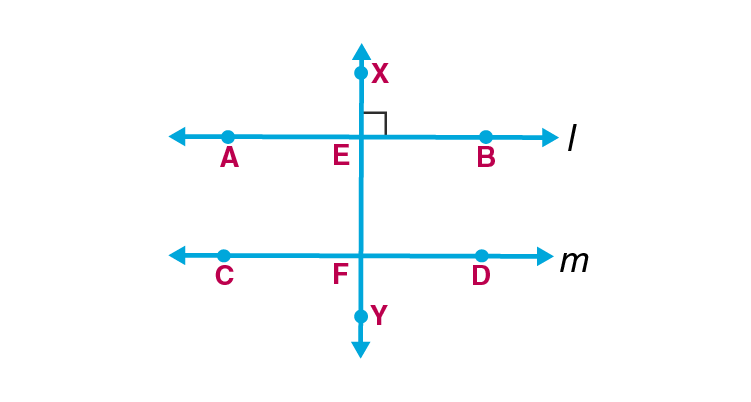Given : Line ABline CD.

Line XY intersects them at E and F respectively.

Line XY line AB

To prove: Line XY line CD

Proof:

Given line XY line AB

XEB = 90˚….(i)

Line ABline CD and XY is the transversal.

XEB = EFD ….(ii) [corresponding angles]

EFD = 90˚ [From (i) and (ii)]

line XY line CD

Hence proved.

4. In figure 2.24, measures of some angles are shown. Using the measures find the measures of x and y and hence show that line l || line m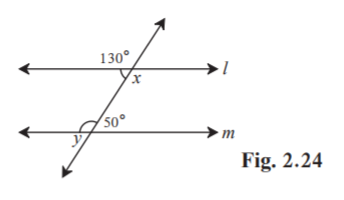Solution:

Proof:

x = 130˚ [x and 130 ˚vertically opposite angles]

y = 50˚ [y and 50 ˚vertically opposite angles]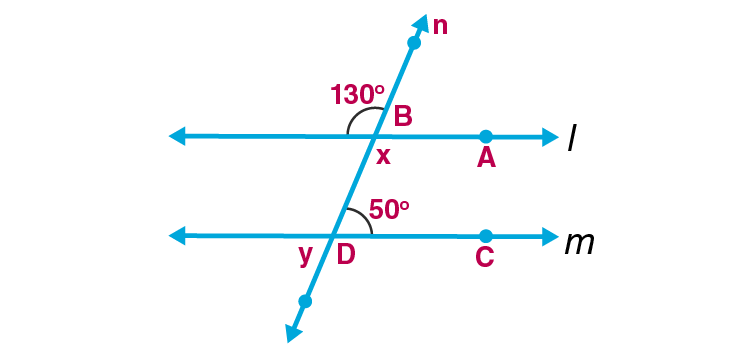Here ABD +CDB = 130+50 = 180

But, ∠ ABD and ∠CDB are a pair of interior angles on lines l and m when line n is the transversal.

line lline m [Interior angles test]

Hence proved.

5. Line AB || line CD || line EF and line QP is their transversal. If y : z = 3 : 7 then find the measure of x. (See figure 2.25.)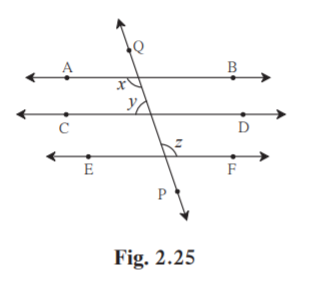Solution:

Given y:z = 3:7

Let k be a common multiple.

y = 3k

z = 7k……(i)

Given line AB line EF.

PQ is the transversal.

x = z [Alternate interior angles]

x = 7k…..(ii)

Given line AB line CD.

PQ is the transversal.

x+y = 180˚ [Interior angles on same side of transversal are supplementary]

7k+3k = 180

10k = 180

k = 180/10 = 18

x = 7k [From (ii)]

= 7×18 = 126˚

Hence measure of x is 126˚.

6. In figure 2.26, if line q || line r, line p is their transversal and if a = 80° find the values of f and g.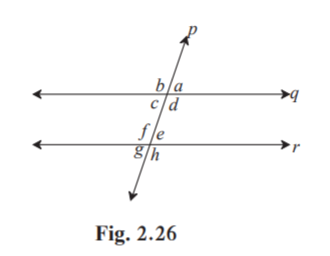Solution:

Given line qline r.

Line p is the transversal.

a = 80˚

c = a = 80˚ [a and c are vertically opposite angles]

f +c = 180 [f and c are interior angles on same side of transversal]

f = 180-80 = 100˚

g +f = 180 [Linear pair]

g = 180-100 = 80˚

Hence measure of f and g are 100˚ and 80˚ respectively.

7. In figure 2.27, if line AB || line CF and line BC || line ED then prove that ABC = FDE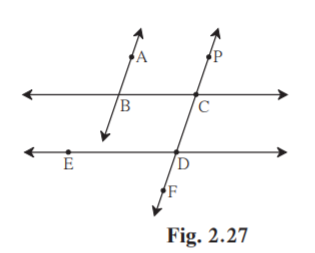Solution:

Given: Line AB line CF and BC is their transversal.

To prove : ABC = FDE

Proof:

Given line AB line CF and BC is their transversal.

ABC = DCB ….(i) [Alternate interior angles]

Given line BC line ED and CD is their transversal.

DCB = FDE….(ii) [Corresponding angles]

ABC = FDE [from (i) and (ii)]

Hence proved.

8. In figure 2.28, line PS is a transversal of parallel line AB and line CD. If Ray QX, ray QY, ray RX, ray RY are angle bisectors, then prove that QXRY is a rectangle.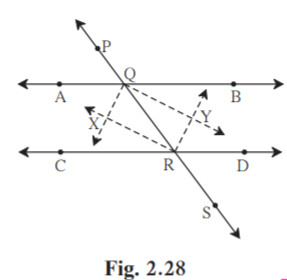Solution:

Given PS is a transversal of parallel line AB and CD.

Ray QX, ray QY, ray RX, ray RY are angle bisectors.

To Prove: QXRY is a rectangle.

Proof: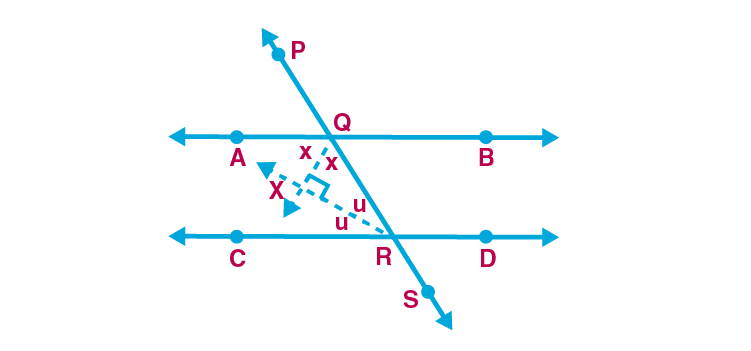XQA = XQR = x°…(i) [Ray QX bisects AQR]

YQR = YQB = y°….(ii) [Ray QY bisects BQR]

XRQ = XRC = u° ….. (iii) [Ray RX bisects CRQ]

YRQ = YRD = v° …(iv) [Ray RY bisects DRQ]

line AB line CD .

Line PS is their transversal.

AQR+ CRQ= 180° [Interior angles on same side of transversal]

AQR = XQA+XQR [Angle addition property]

CRQ = XRC+XRQ [Angle addition property]

(XQA+XQR)+(XRC +XRQ) = 180°

(x+x)+(u+u) = 180° [From (i) and (ii)]

2x+2u = 180°

2(x+u) = 180°

x+u = 90° ……..(v)

In XQR

XQR +XRQ+QXR = 180° [Angle sum property of triangle]

x+u +QXR = 180° [From (i) and (iii)]

90 + QXR = 180° [From (v)]

QXR = 180°-90°

QXR = 90° …..(vi)

Similarily we can prove that,

y+v = 90°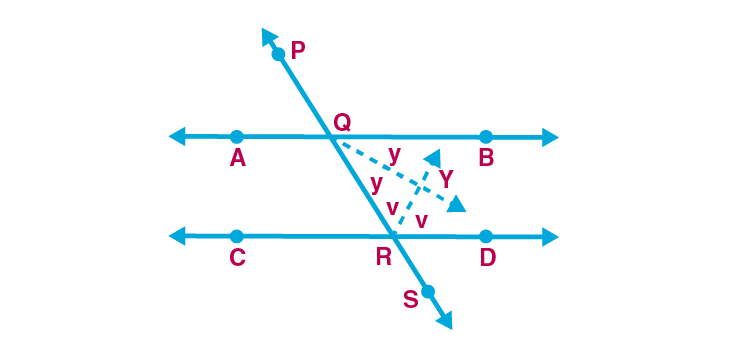Hence QYR = 90° ……(vii)

Now, AQR+BQR = 180° [Linear pair]

AQR = XQA+XQR [Angle addition property]

BQR = YQR+YQB [Angle addition property]

(XQA +XQR)+(YQR+YQB) = 180°

(x+x)+(y+y) = 180° [From (i) and (ii)]

2x+2y = 180°

2(x+y) = 180°

x+y = 90°

i.e. XQR +YQR = 90° [From (i) and (ii)]

XQY = 90° ….(viii) [Angle addition property]

Similarly we can prove that,

XRY = 90° …(ix)

In QXRY

QXR = QYR = XQY = XRY = 90° [From (vi), (vii), (viii) and (ix)]

QXRY is a parallelogram with all angles are right angles.

Rectangle is a parallelogram in which all angles are right angles.

QXRY is a rectangle.

Hence proved.

This chapter also deals with interior angles test, alternate angles test and corresponding angles test. Use of properties of parallel lines is explained in this chapter. Log on to BYJU’S to gain more information on the concepts of Parallel Lines.

## Frequently Asked Questions on Maharashtra State Board Solutions for Class 9 Maths Part 2 Chapter 2 Parallel Lines

### Are these Maharashtra State Board Class 9 Maths Part 2 Chapter 2 Solutions important?

Yes they are very important. These solutions are devised in such a way that  designed in a way that every student can easily grasp the concept into their minds and clarify whatever doubts they have about the topic. The solutions provided are self-explanatory and the step by step guidance will help the students. Also, the board exam aspirants can assess their learning abilities and exam preparations with these solutions.